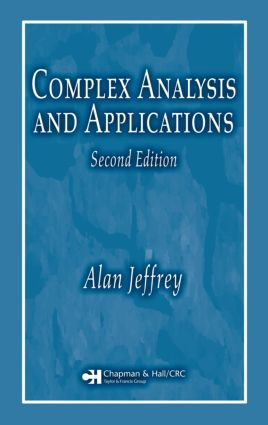# Complex Analysis and Applications

## 2nd Edition

Chapman and Hall/CRC

592 pages | 204 B/W Illus.

Paperback: 9781584885535
pub: 2005-11-10
SAVE ~\$24.00
\$120.00
\$96.00
x
eBook (VitalSource) : 9780429114748
pub: 2005-11-10
from \$57.50

FREE Standard Shipping!

### Description

Complex Analysis and Applications, Second Edition explains complex analysis for students of applied mathematics and engineering. Restructured and completely revised, this textbook first develops the theory of complex analysis, and then examines its geometrical interpretation and application to Dirichlet and Neumann boundary value problems.

A discussion of complex analysis now forms the first three chapters of the book, with a description of conformal mapping and its application to boundary value problems for the two-dimensional Laplace equation forming the final two chapters. This new structure enables students to study theory and applications separately, as needed.

In order to maintain brevity and clarity, the text limits the application of complex analysis to two-dimensional boundary value problems related to temperature distribution, fluid flow, and electrostatics. In each case, in order to show the relevance of complex analysis, each application is preceded by mathematical background that demonstrates how a real valued potential function and its related complex potential can be derived from the mathematics that describes the physical situation.

### Reviews

“This book is an excellent textbook, well written, and enjoyable. It is warmly recommended to students of applied mathematics and engineering that are interested in various applications of complex analysis.”

— Gabriela Kohr, writing in Zentralblatt MATH, Vol. 1113, 2007

### Analytic Functions

Review of Complex Numbers

Curves, Domains, and Regions

Analytic Functions

The Cauchy-Riemann Equations: Proof and Consequences

Elementary Functions

### Complex Integration

Contours and Complex Integrals

The Cauchy Integral Theorem

Antiderivatives and Definite Integrals

The Cauchy Integral Formula

The Cauchy Integral Formula for Derivatives

Useful Results Deducible from the Cauchy Integral Formulas

Evaluation of Improper Integrals by Contour Integration

### Taylor and Laurent Series: Residue Theorem and

Applications

Sequences, Series, and Convergence

Uniform Convergence

Power Series

Taylor Series

Laurent Series

Classification of Singularities and Zeros

Residues and the Residue Theorem

Applications of the Residue Theorem

The Laplace Inversion Integral

### Conformal Mapping

Geometrical Aspects of Analytic Functions: Mapping

Conformal Mapping

The Linear Fractional Transformation

Mappings by Elementary Functions

The Schwarz-Christoffel Transformation

### Boundary Value Problems, Potential Theory, and

Conformal Mapping

Laplace’s Equation and Conformal Mapping – Boundary

Value Problems

Standard Solutions of the Laplace Equation

Two-Dimensional Electrostatics